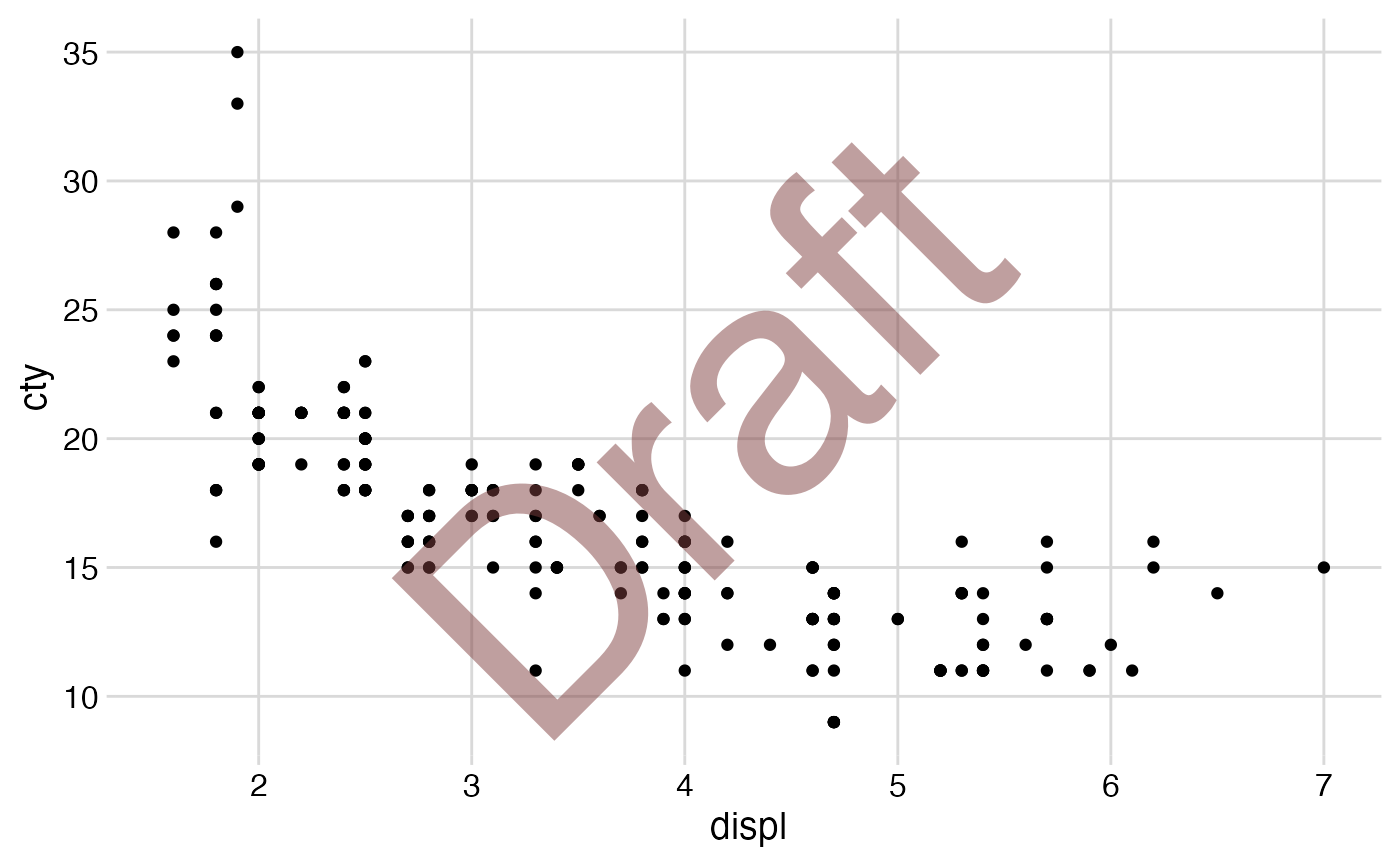Set up a drawing layer on top of a ggplot.

ggdraw(plot = NULL, xlim = c(0, 1), ylim = c(0, 1), clip = "off")

## Arguments

plot The plot to use as a starting point. Can be a ggplot2 plot, an arbitrary grob or gtable, or a recorded base-R plot, as in as_grob(). The x-axis limits for the drawing layer. The y-axis limits for the drawing layer. Should drawing be clipped to the set limits? The default is no ("off").

## Examples

library(ggplot2)

p <- ggplot(mpg, aes(displ, cty)) +
geom_point() +
theme_minimal_grid()
ggdraw(p) + draw_label("Draft", colour = "#80404080", size = 120, angle = 45)